# Fitting models with SITAR

## Introduction

SITAR is a form of growth curve analysis that models the biology of growth. To illustrate this we use the berkeley dataset to explore the growth of height in girls during puberty. If necessary, first install the sitar library.

if (!require(sitar)) install.packages('sitar')

Next load the sitar library, which contains the berkeley dataset, and then fit a SITAR model to the heights of girls from 8 to 18 years.

library(sitar)
ff <- na.omit(berkeley[berkeley$sex == 2 & berkeley$age >= 8 & berkeley\$age <= 18,
c('id', 'age', 'height')])
fh1 <- sitar(x = age, y = height, id = id, data = ff, df = 5)

Next plot the data, before and after SITAR adjustment.

par(mar = c(4,4,1,1) + 0.1, cex = 0.8)
mplot(x = age, y = height, id = id, data = ff, col = id, las = 1)
plot(fh1, opt = 'a', col = id, las = 1, xlim = xaxsd(), ylim = yaxsd())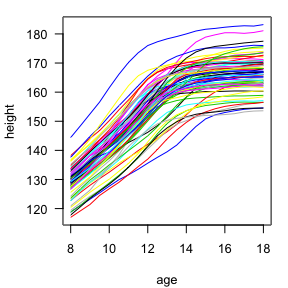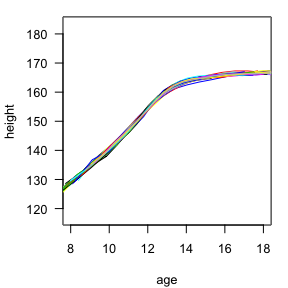The girls were measured every six months, and their heights are shown (left) plotted as growth curves using different colours to separate them. The curves after SITAR adjustment are also shown (right, as discussed below).

## Patterns of growth

Looking at the individiual curves, some girls are consistently taller than average and others consistently shorter. Also some start relatively short and become taller, while others do the opposite. In addition all the girls have a pubertal growth spurt, a time when they grow appreciably faster than before or after, and the timing of the spurt varies between 10 and 14 years.

These are the three growth patterns that SITAR focuses on, which are labelled size, timing and intensity:

• Size is an individual’s mean height compared to the average (measured in cm)
• Timing is the individual’s age at peak height velocity (i.e. the age when they are growing fastest) compared to the average (measured in years)
• Intensity is their growth rate compared to the average (measured as a fraction or percentage)

The SITAR model estimates size, timing and intensity for each individual as random effects, and then uses the values to adjust individual curves to the average. The graph (above right) shows the girls’ curves after they have been SITAR-adjusted, and they are now all superimposed - most of the inter-individual variability has been removed.

This explains the name SITAR - SuperImposition by Translation And Rotation. The curve adjustment involves translatiion (shifting the curves up/down and left/right) and rotation (making them steeper or shallower), and the effect is to superimpose the curves.

## Mean curve

The SITAR mean growth curve is estimated by taking the adjusted curves (above right) and fitting a natural cubic spline through them (below left). The mean height velocity curve, calculated as the first derivative of the mean curve, is also shown (right, dashed). The mean age at peak height velocity is 11.7 years, marked by the vertical dotted line in the plots, and the mean peak height velocity is 7.8 cm/year.

The complexity of the spline curve’s shape is determined by its number of degrees of freedom, as set with the df argument in the sitar call. Here there are five degrees of freedom (see first code chunk).

par(mar = c(4,4,1,1) + 0.1, cex = 0.8)
plot(fh1, opt = 'd', las = 1, apv = TRUE)
plot(fh1, opt = 'v', las = 1, apv = TRUE, lty = 2)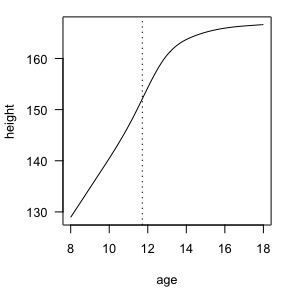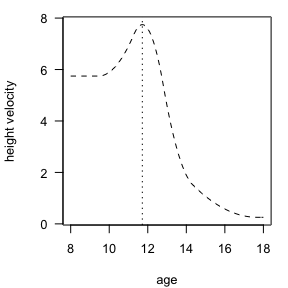## Size, timing and intensity

To see the effect of SITAR adjustment in individual curves, the plot (below left) shows all the curves in gray as background, plus curves for the tallest and shortest girls before and after adjustment, along with the mean curve (shown dashed). For the tall girl (id 310 in blue), her mean height is estimated as 14.8 cm greater than average (size), her age at peak height velocity is 1.4 years earlier than average (timing), and her mean growth rate is 25% faster than average (intensity). Her adjusted curve, which incorporates these differences, appears as the blue curve close to the mean curve.

par(mar = c(4,4,1,1) + 0.1, cex = 0.8)
plot(fh1, opt = 'u', las = 1, col = 8, lwd = 0.5)
lines(fh1, opt = 'd', lty = 2)
lines(fh1, opt = 'ua', col = id, subset = id == 310 | id == 355)
legend('bottomright', c('id 310', 'mean', 'id 355'), lty = c(1, 2, 1), col = c(4, 1, 2), cex = 0.8, inset=0.04)
pairs(ranef(fh1), labels = c('size', 'timing', 'intensity'), pch=20)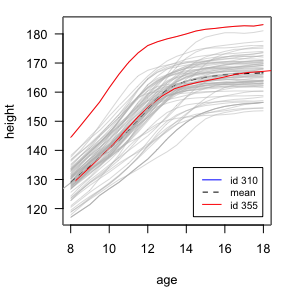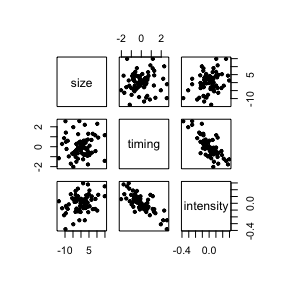Correspondingly the short girl (id 355 in red) is 9.5 cm shorter, 1.4 years later and 15% slower growing than average. Her adjusted curve is the upper red curve. Note that the two adjusted curves are very close to the mean curve, showing how effectively SITAR superimposes the curves.

The random effects of size, timing and intensity are accessed using the random.effects() or ranef() function. The associations between the random effects are shown in the scatterplot (above right), where timing is negatively correlated with intensity, but size is uncorrelated with timing and intensity. The corresponding correlation matrix is shown below, with the random effects labelled a, b and c respectively. So early puberty is associated with faster growth – and hence a shorter growth spurt.

a b c
a 1.00 0.08 0.31
b 0.08 1.00 -0.77
c 0.31 -0.77 1.00

Here are the values of the random effects for the first ten girls, including id 310, where the intensity c, multiplied by 100, is a percentage.

a b c
301 -7.65 -0.98 -0.02
302 -0.25 0.20 -0.05
303 -4.78 -2.03 0.06
304 1.51 0.19 -0.07
305 4.29 0.72 -0.05
306 -1.84 -0.08 0.10
307 0.84 -0.12 0.03
308 -1.02 2.57 -0.25
309 -2.78 0.29 -0.08
310 14.83 -1.43 0.25

## Biology of SITAR

The SITAR model relies on the concept of developmental age, and it assumes that chronological age and developmental age in individual children are linearly related. An individual may be advanced or delayed in age terms, which is reflected in their timing parameter, and they may be becoming more or less advanced over time, as flagged by their intensity parameter. Thus the analysis models on both the height scale (i.e. the size parameter) and the age scale, and in this sense it mimics biology as the appropriate age scale is developmental age not chronological age.

## Algebra of SITAR

The algebra underlying the SITAR model is as follows: $y_{ij} = \alpha_i + h[\frac{t_{ij} - \beta_i}{exp(-\gamma_i)}] + \epsilon_{ij}$ where $$y_{ij}$$ and $$t_{ij}$$ are height and age, and $$\epsilon_{ij}$$ is the residual, for subject $$i$$ and measurement $$j$$; $$h[.]$$ is a natural cubic spline function, and $$\alpha_i$$, $$\beta_i$$ and $$\gamma_i$$ are respectively the size, timing and intensity parameters for subject $$i$$.